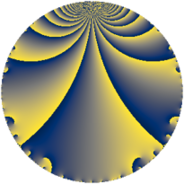# Properties

 Label 3675.2.a.nLevel $3675$ Weight $2$ Character orbit 3675.a Self dual yes Analytic conductor $29.345$ Analytic rank $1$ Dimension $1$ CM no Inner twists $1$

# Related objects

## Newspace parameters

 Level: $$N$$ $$=$$ $$3675 = 3 \cdot 5^{2} \cdot 7^{2}$$ Weight: $$k$$ $$=$$ $$2$$ Character orbit: $$[\chi]$$ $$=$$ 3675.a (trivial)

## Newform invariants

 Self dual: yes Analytic conductor: $$29.3450227428$$ Analytic rank: $$1$$ Dimension: $$1$$ Coefficient field: $$\mathbb{Q}$$ Coefficient ring: $$\mathbb{Z}$$ Coefficient ring index: $$1$$ Twist minimal: no (minimal twist has level 21) Fricke sign: $$1$$ Sato-Tate group: $\mathrm{SU}(2)$

## $q$-expansion

 $$f(q)$$ $$=$$ $$q + q^{2} + q^{3} - q^{4} + q^{6} - 3q^{8} + q^{9} + O(q^{10})$$ $$q + q^{2} + q^{3} - q^{4} + q^{6} - 3q^{8} + q^{9} + 4q^{11} - q^{12} - 2q^{13} - q^{16} - 6q^{17} + q^{18} - 4q^{19} + 4q^{22} - 3q^{24} - 2q^{26} + q^{27} - 2q^{29} + 5q^{32} + 4q^{33} - 6q^{34} - q^{36} - 6q^{37} - 4q^{38} - 2q^{39} - 2q^{41} + 4q^{43} - 4q^{44} - q^{48} - 6q^{51} + 2q^{52} - 6q^{53} + q^{54} - 4q^{57} - 2q^{58} - 12q^{59} + 2q^{61} + 7q^{64} + 4q^{66} - 4q^{67} + 6q^{68} - 3q^{72} - 6q^{73} - 6q^{74} + 4q^{76} - 2q^{78} - 16q^{79} + q^{81} - 2q^{82} - 12q^{83} + 4q^{86} - 2q^{87} - 12q^{88} + 14q^{89} + 5q^{96} + 18q^{97} + 4q^{99} + O(q^{100})$$

## Embeddings

For each embedding $$\iota_m$$ of the coefficient field, the values $$\iota_m(a_n)$$ are shown below.

For more information on an embedded modular form you can click on its label.

Label $$\iota_m(\nu)$$ $$a_{2}$$ $$a_{3}$$ $$a_{4}$$ $$a_{5}$$ $$a_{6}$$ $$a_{7}$$ $$a_{8}$$ $$a_{9}$$ $$a_{10}$$
1.1
 0
1.00000 1.00000 −1.00000 0 1.00000 0 −3.00000 1.00000 0
 $$n$$: e.g. 2-40 or 990-1000 Significant digits: Format: Complex embeddings Normalized embeddings Satake parameters Satake angles

## Atkin-Lehner signs

$$p$$ Sign
$$3$$ $$-1$$
$$5$$ $$1$$
$$7$$ $$-1$$

## Inner twists

This newform does not admit any (nontrivial) inner twists.

## Twists

By twisting character orbit
Char Parity Ord Mult Type Twist Min Dim
1.a even 1 1 trivial 3675.2.a.n 1
5.b even 2 1 147.2.a.a 1
7.b odd 2 1 525.2.a.d 1
15.d odd 2 1 441.2.a.f 1
20.d odd 2 1 2352.2.a.v 1
21.c even 2 1 1575.2.a.c 1
28.d even 2 1 8400.2.a.bn 1
35.c odd 2 1 21.2.a.a 1
35.f even 4 2 525.2.d.a 2
35.i odd 6 2 147.2.e.b 2
35.j even 6 2 147.2.e.c 2
40.e odd 2 1 9408.2.a.m 1
40.f even 2 1 9408.2.a.bv 1
60.h even 2 1 7056.2.a.p 1
105.g even 2 1 63.2.a.a 1
105.k odd 4 2 1575.2.d.a 2
105.o odd 6 2 441.2.e.b 2
105.p even 6 2 441.2.e.a 2
140.c even 2 1 336.2.a.a 1
140.p odd 6 2 2352.2.q.e 2
140.s even 6 2 2352.2.q.x 2
280.c odd 2 1 1344.2.a.g 1
280.n even 2 1 1344.2.a.s 1
315.z even 6 2 567.2.f.b 2
315.bg odd 6 2 567.2.f.g 2
385.h even 2 1 2541.2.a.j 1
420.o odd 2 1 1008.2.a.l 1
455.h odd 2 1 3549.2.a.c 1
560.be even 4 2 5376.2.c.l 2
560.bf odd 4 2 5376.2.c.r 2
595.b odd 2 1 6069.2.a.b 1
665.g even 2 1 7581.2.a.d 1
840.b odd 2 1 4032.2.a.k 1
840.u even 2 1 4032.2.a.h 1
1155.e odd 2 1 7623.2.a.g 1

By twisted newform orbit
Twist Min Dim Char Parity Ord Mult Type
21.2.a.a 1 35.c odd 2 1
63.2.a.a 1 105.g even 2 1
147.2.a.a 1 5.b even 2 1
147.2.e.b 2 35.i odd 6 2
147.2.e.c 2 35.j even 6 2
336.2.a.a 1 140.c even 2 1
441.2.a.f 1 15.d odd 2 1
441.2.e.a 2 105.p even 6 2
441.2.e.b 2 105.o odd 6 2
525.2.a.d 1 7.b odd 2 1
525.2.d.a 2 35.f even 4 2
567.2.f.b 2 315.z even 6 2
567.2.f.g 2 315.bg odd 6 2
1008.2.a.l 1 420.o odd 2 1
1344.2.a.g 1 280.c odd 2 1
1344.2.a.s 1 280.n even 2 1
1575.2.a.c 1 21.c even 2 1
1575.2.d.a 2 105.k odd 4 2
2352.2.a.v 1 20.d odd 2 1
2352.2.q.e 2 140.p odd 6 2
2352.2.q.x 2 140.s even 6 2
2541.2.a.j 1 385.h even 2 1
3549.2.a.c 1 455.h odd 2 1
3675.2.a.n 1 1.a even 1 1 trivial
4032.2.a.h 1 840.u even 2 1
4032.2.a.k 1 840.b odd 2 1
5376.2.c.l 2 560.be even 4 2
5376.2.c.r 2 560.bf odd 4 2
6069.2.a.b 1 595.b odd 2 1
7056.2.a.p 1 60.h even 2 1
7581.2.a.d 1 665.g even 2 1
7623.2.a.g 1 1155.e odd 2 1
8400.2.a.bn 1 28.d even 2 1
9408.2.a.m 1 40.e odd 2 1
9408.2.a.bv 1 40.f even 2 1

## Hecke kernels

This newform subspace can be constructed as the intersection of the kernels of the following linear operators acting on $$S_{2}^{\mathrm{new}}(\Gamma_0(3675))$$:

 $$T_{2} - 1$$ $$T_{11} - 4$$ $$T_{13} + 2$$

## Hecke characteristic polynomials

$p$ $F_p(T)$
$2$ $$-1 + T$$
$3$ $$-1 + T$$
$5$ $$T$$
$7$ $$T$$
$11$ $$-4 + T$$
$13$ $$2 + T$$
$17$ $$6 + T$$
$19$ $$4 + T$$
$23$ $$T$$
$29$ $$2 + T$$
$31$ $$T$$
$37$ $$6 + T$$
$41$ $$2 + T$$
$43$ $$-4 + T$$
$47$ $$T$$
$53$ $$6 + T$$
$59$ $$12 + T$$
$61$ $$-2 + T$$
$67$ $$4 + T$$
$71$ $$T$$
$73$ $$6 + T$$
$79$ $$16 + T$$
$83$ $$12 + T$$
$89$ $$-14 + T$$
$97$ $$-18 + T$$